# Constrained Nonlinear Optimization VI

## LabVIEW 2018 Help

Edition Date: March 2018
Part Number: 371361R-01
View Product InfoLabVIEW 2016 HelpLabVIEW 2017 HelpLabVIEW 2018 HelpLabVIEW 2019 HelpLabVIEW 2020 Help

Owning Palette: Optimization VIs

Requires: Full Development System

Solves a general nonlinear optimization problem with nonlinear equality constraint and nonlinear inequality constraint bounds using a sequential quadratic programming method.

Example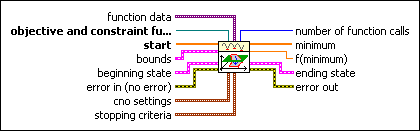function data contains static data that the user-defined function needs at run time.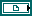objective and constraint function is a reference to the VI that implements the nonlinear function to minimize, the nonlinear equality constraints function, and the nonlinear inequality constraints function as separate outputs. The objective function output must not be empty. The constraint functions are optional. Therefore, the equality constraints output, the inequality constraints output, or both can be empty.

Create this VI by starting from the VI template located in labview\vi.lib\gmath\NumericalOptimization\cno_objective function template.vit.start are the points in n dimension at which the optimization process starts.bounds is a cluster that contains the upper and lower numeric limits for the parameters being optimized and the inequality constraints.X minimum contains the lowest allowed value of the parameters being optimized. The length of this array must match the length of X maximum and can be 0 or the same as the length of the start array. The array does not accept exceptional values, such as Inf, –Inf, or NaN.X maximum contains the highest allowed value of the parameters being optimized. The length of this array must match the length of X minimum and can be 0 or the same as the length of the start array. The array does not accept exceptional values, such as Inf, –Inf, or NaN.inequality constraint minimum contains the lowest allowed value of the inequality constraints. The length of this array must match the length of the inequality constraints returned from the objective and constraint function. This array does not accept exceptional values, such as Inf, –Inf, or NaN.inequality constraint maximum contains the highest allowed value of the inequality constraints. The length of this array must match the length of the inequality constraints returned from the objective and constraint function. This array does not accept exceptional values, such as Inf, –Inf, or NaN.beginning state contains the initial values of the inequality constraint function, the Lagrangian multipliers, and the Hessian. beginning state is typically the ending state of a previous optimization and allows a warm start of the optimization.inequality constraints contains the value of the inequality constraint functions, typically from a previous call to the Constrained Nonlinear Optimization VI.lagrangian multipliers contains the value of the Lagrangian multipliers, typically from a previous call to the Constrained Nonlinear Optimization VI.hessian contains an estimate of the Hessian, typically from a previous call to the Constrained Nonlinear Optimization VI.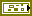error in describes error conditions that occur before this node runs. This input provides standard error in functionality.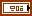cno settings contains additional tolerance and termination settings that are specific to this algorithm.constraint weight is a relative weighting of the constraints as compared to the objective function and controls the distance that the optimization search travels outside the feasible range to seek an optimal solution. A value greater than 1 forces the search to stay close to the known feasible range, and a smaller value allows a broader range of searching.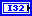maximum minor iterations is an upper bound on the number of iterations allowed to solve the quadratic problem. If the constraint functions are highly nonlinear, the linearized constraints used in the quadratic problem might not be very accurate. In this case, it might be sensible to limit the number of minor iterations, forcing the linear approximation to be updated more often.stopping criteria is the collection of conditions that terminate the optimization. If (function tolerance AND parameter tolerance AND gradient tolerance) OR max iterations OR max function calls then optimization terminates.function tolerance is the relative change in function value and is defined as abs(current f – prev f)/(abs(curr f)+machine eps). If the relative change in the function value falls below function tolerance, the optimization terminates.parameter tolerance is the relative change in parameter values and is defined as abs(current p – prev p)/(abs(curr p)+machine eps). If the relative change of all the parameter values falls below parameters tolerance, the optimization terminates.gradient tolerance is the 2–norm of the gradient. If the 2–norm of the gradient falls below gradient tolerance, the optimization terminates.max iterations is the largest number of iterations of the major loop of the optimization. If the number of major loop iterations exceeds max iterations, the optimization terminates.max function calls is the largest number of objective function calls allowed before terminating the optimization process.max time (sec) is the maximum amount of time LabVIEW allows between the start and the end of the optimization process. The default is –1. –1 indicates never to time out.number of function calls is the number of times the objective function calls in the optimization process.

If max function calls is -1, number of function calls equals population size * (max iterations + 1).minimum is the determined local minimum in n dimension.f(minimum) is the function value of objective function at the determined minimum.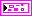ending state contains the final values of the inequality constraint function, the Lagrange multipliers, and the Hessian.inequality constraints contains the value of the inequality constraint functions at the end of the optimization.lagrangian multipliers contains the value of the Lagrangian multipliers at the end of the optimization.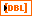hessian contains an estimate of the Hessian at the end of the optimization.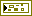error out contains error information. This output provides standard error out functionality.

## Example

Refer to the Nonlinear Spring Constant fit VI in the labview\examples\Mathematics\Fitting directory for an example of using the Constrained Nonlinear Optimization VI.